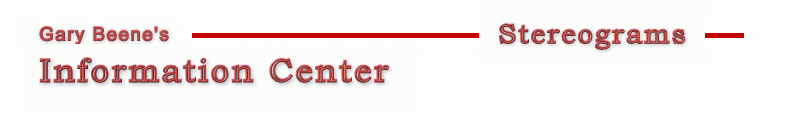Stereogram Algorithms - Oversampling Stereograms created by this basic code are fun to view, but suffer from several visual shortcomings - primarily the apparent layering of the object being viewed. Other visual issues (echoes, artifacts, holes, ...) can also be seen in the results of the basic code. Basic Stereogram with Oversampling Algorithm In this example, two pictureboxes are used - one to provide the depth information (picDepth) and one to display the stereogram (picOut). ```1 Dim x As Long, y As Long, maxX As Long, maxY As Long, same() As Long 'basic 2 Dim z() As Single, mu As Single, E As Long, DPI As Long, s As Long 'basic 3 Dim right As Long, left As Long, w As Single 'basic 4 Dim L As Long 'smart links 5 Dim zt As Single, t As Long, visible As Long 'hidden surface removal 6 Dim R As Long, G As Long, B As Long, i As Long 'oversampling 7 Dim p() As Long, oversample As Long 'oversampling 8 oversample = 2: DPI = 72: E = 2.5 * DPI * oversample: mu = 0.2: w = 0.5 9 maxX = picDepth.ScaleWidth * oversample: maxY = picDepth.ScaleHeight 10 ReDim same(maxX), z(maxX, maxY), p(maxX, maxY): Randomize 11 For y = 0 To maxY 12 For x = 0 To maxX - 1 '1 scanline 13 z(x, y) = picDepth.Point(x / oversample, y) / 16777215 'initialize 14 same(x) = x 'initialize 15 s = (1 - mu * z(x, y)) * E / (2 - mu * z(x, y)) 'image plane separation 16 left = x - (s / 2) 'left link position 17 right = left + s 'right link position 18 If 0 <= left And right < maxX Then same(left) = right 'create link 19 Next x 20 For x = maxX - 1 To 0 Step -1 'set colors 21 If same(x) = x Then 22 p(x, y) = Int(Rnd + w) * vbWhite 'Rnd*vbwhite for color 23 Else 24 p(x, y) = p(same(x), y) 'link color 25 End If 26 Next x 27 Next y 28 For y = 0 To maxY: For x = 0 To maxX - 1 Step oversample 'average pixel colors 29 R = 0: G = 0: B = 0 30 For i = 0 To oversample - 1 31 R = R + p(x + i, y) Mod 256 32 G = G + (p(x + i, y) \ 256) Mod 256 33 B = B + (p(x + i, y) \ 256 \ 256) Mod 256 34 Next i 35 picOut.PSet (x / oversample, y), RGB(R / oversample, G / oversample, B / oversample) 36 Next x: Next y ```